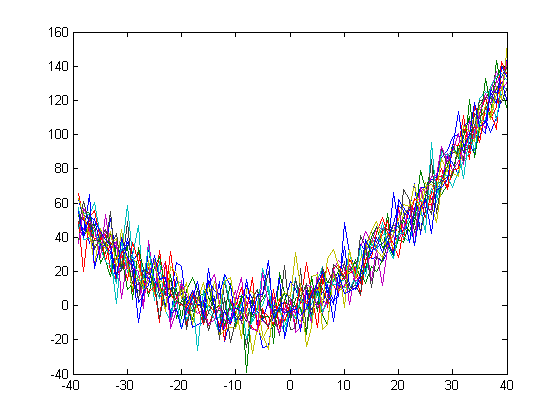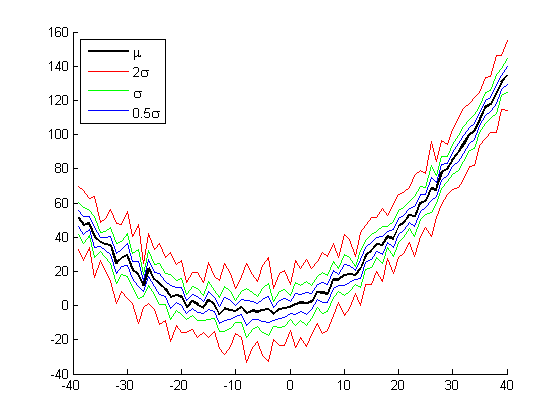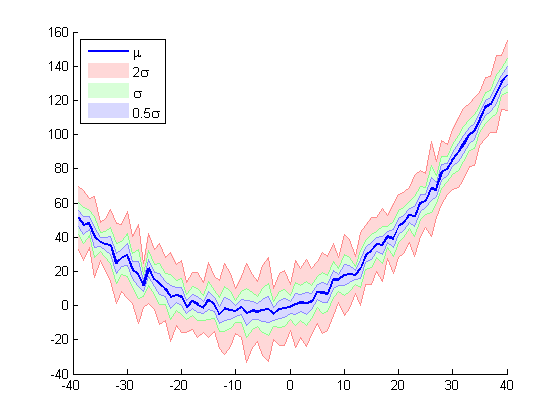## File Exchange Pick of the WeekOur best user submissions

Jiro's pick this week is shadedErrorBar by Rob Campbell.

This one was suggested to us by Oliver, but I'm pretty certain I would have picked it if I had come across this entry.

In fact, we used to (try to) make similar plots back in my graduate school days. We had some time series data from multiple subjects, and we wanted a way to see trends and variability. It took a little while for me to arrive at this way of visualizing variability. Initially, I would simply plot the line series and visually inspect how close or separated the lines were. We already had quantitative measures, but I also wanted the visual representation.

% From one of Rob's examples
x=(1:80)-40;
y=ones(15,1)*x; y=y+0.06*y.^2+randn(size(y))*10;

plot(x, y);Next, I simply created lines to represent the mean and multiple standard deviations. This was better, but it was still a bit crowded visually.

mean_y = mean(y);
std_y = std(y);

figure;hold on;

H1 = plot(x, mean_y, 'Color', 'k', 'LineWidth', 2);
H2 = plot(x, [mean_y - 2*std_y; mean_y + 2*std_y], 'Color', 'r');
H3 = plot(x, [mean_y - std_y; mean_y + std_y], 'Color', 'm');
H4 = plot(x, [mean_y - 0.5*std_y  ; mean_y +   0.5*std_y], 'Color', 'b');

legend([H1, H2(1), H3(1), H4(1)], ...
'\mu', '2\sigma', '\sigma', '0.5\sigma', ...
'Location', 'Northwest');Finally, we decided on a shaded representation of bounds. I don't remember how complicated the code was, but with Rob's shadedErrorBar, I can do this very easily!

figure;hold on;

H(1) = shadedErrorBar(x, y, {@mean, @(x) 2*std(x)  }, '-r', 0);
H(2) = shadedErrorBar(x, y, {@mean, @(x) 1*std(x)  }, '-m', 0);
H(3) = shadedErrorBar(x, y, {@mean, @(x) 0.5*std(x)}, {'-b', 'LineWidth', 2}, 0);

legend([H(3).mainLine, H.patch], ...
'\mu', '2\sigma', '\sigma', '0.5\sigma', ...
'Location', 'Northwest');The code is well-written, with help text, examples, error-checking, and lots of comments – everything that I look for in a good MATLAB code! Thanks for your entry, Rob, and thanks Oliver for the suggestion!